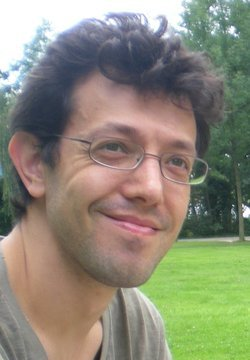# Assaf Naor

## 2012 Regional Award Winner — FacultyCurrent Position:
Professor of Mathematics

Institution:
Princeton University (Formerly at the Courant Institute of Mathematical Sciences, New York University)

Discipline:
Mathematics & Computer Science

Recognized for: Analysis, probability, quantitative geometry, linear and non-linear geometric Banach space theory, structure theory of metric spaces, and their applications to theoretical computer science, combinatorics and mathematical physics

Areas of Research Interest and Expertise: Analysis and quantitative geometry, and their applications to approximation algorithms.

Biography:

• PhD, Mathematics, Hebrew University, Jerusalem
• BS, Mathematics, Hebrew University, Jerusalem

Assaf Naor’s research focuses on analysis, probability, quantitative geometry and their applications to combinatorics, mathematical physics and theoretical computer science.  Before coming to New York University, he was a member of the Theory Group at Microsoft Research.

Developing a structure theory for metric spaces that is motivated by the search for a “dictionary” that relates very general geometries to simpler linear spaces, allowing one to apply deep insights from the linear theory to uncover hidden structures in situations which do not have any a priori link to linear spaces. An example of such a structural result is the ``Ultrametric Skeleton Theorem.” Such general results have a wide variety of applications in pure mathematics as well as in theoretical computer science. For example, the above research program leads to the best known algorithms and impossibility results for the fundamental Sparsest Cut problem in the field of approximation algorithms. Additional applications of high-dimensional geometry to algorithm design arise from work on Grothendieck inequalities, including a recent new higher dimensional binary rounding method that yields the first improved upper bound on the classical Grothendieck constant since 1977.

In 2012 Dr. Naor became a Fellow of the American Mathematical Society and in 2013 he was invited to give the Minvera Lectures at Princeton University's Department of Mathematics.

Since January 2014, Dr. Naor is leading the Simons Foundation’s Collaboration on Algorithms and Geometry, a program intended to address deep questions at the interface of mathematics and theoretical computer science.

"My goal is to further the understanding of basic geometric structures that permeate mathematics, computer science, and the sciences in general. Such a foundational understanding is important in its own right, but in addition, due to the ubiquity of the geometries to which it applies, it yields a powerful and versatile tool in a wide-variety of algorithmic contexts. "

Key Publications:

1. Mendel M, Naor A., Metric cotypeAnnals of Mathematics. 2008
2. Arora S, Lee J, Naor A., Euclidean distortion and the sparsest cutJournal of the American Mathematical Society. 2008
3. Mark B, Konstantin M, Yury M, Assaf N., The Grothendieck constant is strictly smaller than Krivine’s bound. Forum of Mathematics, Pi, Volume 1/2013, e4. An extended abstract appeared in FOCS, 2011

Other Honors:

2011 Pazy Memorial Research Award
2011 Bôcher Memorial Prize
2008 European Math Society Prize
2008 Salem Prize

In the Media:

Simons Foundation Launches Simons Collaboration on Algorithms and Geometry. Simons Foundation. January 16, 2014